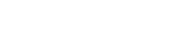# height

The desired height of the output image.

The `height` parameter enables dynamic height resizing based on pixels and percent values.

The `{value}` can be either a positive integer or a positive number less than 1. If the value is an integer greater than or equal to 1, the value is interpreted as a pixel height. If the height parameter is less than 1, the value is interpreted as a percentage height.

Unless a `width` parameter is present, the `height` value will cause the width of the image to be scaled in proportion to the requested height. If both width and height are omitted, the input image dimensions are used.

## Syntax

``height={value}``

`value` may be one of:

• Absolute height: An integer number of pixels.
• Relative height: A fraction between 0 and 0.99 (e.g., `0.5`) or a percentage between 0 and 100 followed by the letter `p` (e.g., `50p`). In either case, the value indicates the desired height relative to the image's natural height.
1. The maximum output dimensions are 8,192 x 8,192 pixels.
2. Because we use a positive number between `0` and `0.99` to return a percentage-based scaled image, in order to return an image that is 100% or more, a `p` suffix can be appended to the value. For example, `height=250p` would return an image that is `250%` the height of the original. We use `p` as a simple way to represent percentages.
3. If the requested height is not a whole number of pixels (e.g., as a result of applying a percentage value for `height`), it is rounded to the nearest integer.

## Examples

Click the links to view the transformed image using a demo Fastly IO service.

Example usageDescription
`?height=200`Resize the height to 200px
`?height=0.10`Resize the height to 10% of the input image
`?height=150p`Resize the height to 150% of the input image
`?height=200&width=0.50`Force resize to a height of 200px and a width that is 50% of the original
`?height=150&width=150&fit=bounds`Fit image within a 150 x 150px rectangle
`?height=200&dpr=2`Resize the height to 400px (200 CSS pixels for screens with a device pixel ratio of 2)

BETA